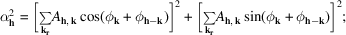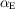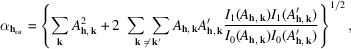International
Tables for
Crystallography
Volume B
Reciprocal space
Edited by U. Shmueli

International Tables for Crystallography (2010). Vol. B, ch. 2.5, pp. 391-392   | 1 | 2 |

## Section 2.5.8.4. The tangent formula

D. L. Dorsete*

#### 2.5.8.4. The tangent formula

| top | pdf |

Given a triple phase relationshipwhere h, k andform a vector sum, it is often possible to find a more reliable estimate ofwhen all the possible vectorial contributions to it within the observed data setare considered as an average, viz:For actual phase determination, this can be formalized as follows. After calculating normalized structure-factor magnitudesfrom the observedto generate all possible phase triples within a reasonably highthreshold, new phase values can be estimated after origin definition by use of the tangent formula (Karle & Hauptman, 1956):The reliability of the phase estimate depends on the variance, which is directly related to the magnitude of, i.e.is identical to the A value defined in the previous section. In the initial stages of phase determinationis replaced by an expectation valueuntil enough phases are available to permit its calculation.

The phase solutions indicated by the tangent formula can thus be ranked according to the phase variance and the determination of phases can be made symbolically from the most probable triple-product relationships. This procedure is equivalent to the one described above for the evaluation of phase-invariant sums by symbolic addition. This procedure may allow determination of a large enough basis phase set to produce an interpretable map.

An alternative procedure is to use an automatic version of the tangent formula in a multisolution process. This procedure is described in Chapter 2.2. After origin definition, enough algebraic unknowns are defined (two values if centrosymmetric and four values, cycling through phase quadrants, if noncentrosymmetric) to access as many of the unknown phases as possible. These are used to generate a number of trial phase sets and the likelihood of identifying the correct solution is based on the use of some figure of merit.

Multisolution approaches employing the tangent formula include MULTAN (Germain et al., 1971), QTAN (Langs & DeTitta, 1975) and RANTAN (Yao, 1981). RANTAN is a version of MULTAN that allows for a larger initial random phase set (with suitable control of weights in the tangent formula). QTAN utilizes thedefinition, wherefor evaluating the phase variance. (Here,are modified Bessel functions.) After multiple solutions are generated, it is desirable to locate the structurally most relevant phase sets by some figure of merit. There are many that have been suggested (Chapter 2.2). The most useful figure of merit in QTAN has been the NQEST (De Titta et al., 1975) estimate of negative quartet invariants (see Chapter 2.2). More recently, this has been superseded by the minimal function (Hauptman, 1993):whereand.

In the first application (Dorset et al., 1979) of multisolution phasing to electron-diffraction data (using the program QTAN), n-beam dynamical structure factors generated for cytosine and disodium 4-oxypyrimidine-2-sulfinate were used to assess the effect of increasing crystal thickness and electron accelerating voltage on the success of the structure determination. At 100 kV samples at least 80 Å thick were usable for data collection and at 1000 kV this sample thickness limit could be pushed to 300 Å – or, perhaps, 610 Å if a partial structure were accepted for later Fourier refinement. NQEST identified the best phase solutions. Later QTAN was used to evaluate the effect of elastic crystal bend on the structure analysis of cytosine (Moss & Dorset, 1982).

In actual experimental applications, two forms of thiourea were investigated with QTAN (Dorset, 1992d), using published three-dimensional electron-diffraction intensities (Dvoryankin & Vainshtein, 1960, 1962). Analysis of the centrosymmetric paraelectric structure yielded results equivalent to those found earlier by symbolic addition (Dorset, 1991b). Analysis of the noncentrosymmetric ferroelectric form was also quite successful (Dorset, 1992d). In both cases, the correct structure was found at the lowest value of NQEST. Re-analysis of the diketopiperazine structure with QTAN also found the correct structure (Dorset & McCourt, 1994a) within the four lowest values of NQEST, but not the one at the lowest value. The effectiveness of this figure of merit became more questionable when QTAN was used to solve the noncentrosymmetric crystal structure of a polymer (Dorset, McCourt, Kopp et al., 1994). The solution could not be found readily when NQEST was used but was easily identified when the minimal functionwas employed instead.

MULTAN has been used to phase simulated data from copper perchlorophthalocyanine (Fan et al., 1985). Within the 2 Å resolution of the electron-microscope image, if one seeks phases for diffraction data in reciprocal-space regions where the objective lens phase contrast transfer function, the method proves to be successful. The method is also quite effective for phase extension from 2 Å to 1 Å diffraction resolution, where the low-angle data serve as a large initial phase set for the tangent formula. However, no useful results were found from an ab initio phase determination carried out solely with the electron-diffraction structure-factor magnitudes. Similar results were obtained when RANTAN was used to phase experimental data from this compound (Fan et al., 1991), i.e. the multisolution approach worked well for phase extension but not for ab initio phase determination. Additional tests were subsequently carried out with QTAN on an experimental hk0 electron-diffraction data set collected at 1200 kV (Dorset, McCourt, Fryer et al., 1994). Again, ab initio phase determination is not possible by this technique. However, if a basis set was constructed from the Fourier transform of a 2.4 Å image, a correct solution could be found, but not at the lowest value of NQEST. This figure of merit was useful, however, when the basis set was taken from the symbolic addition determination mentioned in the previous section.

### References

De Titta, G. T., Edmonds, J. W., Langs, D. A. & Hauptman, H. (1975). Use of negative quartet cosine invariants as a phasing figure of merit: NQEST. Acta Cryst. A31, 472–479.
Dorset, D. L. (1991b). Is electron crystallography possible? The direct determination of organic crystal structures. Ultramicroscopy, 38, 23–40.
Dorset, D. L. (1992d). Automated phase determination in electron crystallography: thermotropic phases of thiourea. Ultramicroscopy, 45, 357–364.
Dorset, D. L., Jap, B. K., Ho, M.-H. & Glaeser, R. M. (1979). Direct phasing of electron diffraction data from organic crystals: the effect of n-beam dynamical scattering. Acta Cryst. A35, 1001–1009.
Dorset, D. L. & McCourt, M. P. (1994a). Automated structure analysis in electron crystallography: phase determination with the tangent formula and least-squares refinement. Acta Cryst. A50, 287–292.
Dorset, D. L., McCourt, M. P., Fryer, J. R., Tivol, W. F. & Turner, J. N. (1994). The tangent formula in electron crystallography: phase determination of copper perchlorophthalocyanine. Microsc. Soc. Am. Bull. 24, 398–404.
Dorset, D. L., McCourt, M. P., Kopp, S., Wittmann, J.-C. & Lotz, B. (1994). Direct determination of polymer crystal structures by electron crystallography – isotactic poly(1-butene), form III. Acta Cryst. B50, 201–208.
Dvoryankin, V. F. & Vainshtein, B. K. (1960). An electron diffraction study of thiourea. Sov. Phys. Crystallogr. 5, 564–574.
Dvoryankin, V. F. & Vainshtein, B. K. (1962). An electron diffraction study of the low-temperature ferroelectric form of thiourea. Sov. Phys. Crystallogr. 6, 765–772.
Fan, H. F., Xiang, S. B., Li, F. H., Pan, Q., Uyeda, N. & Fujiyoshi, Y. (1991). Image resolution enhancement by combining information from electron diffraction pattern and micrograph. Ultramicroscopy, 36, 361–365.
Fan, H.-F., Zhong, Z.-Y., Zheng, C.-D. & Li, F.-H. (1985). Image processing in high-resolution electron microscopy using the direct method. I. Phase extension. Acta Cryst. A41, 163–165.
Germain, G., Main, P. & Woolfson, M. M. (1971). The application of phase relationships to complex structures. III. The optimum use of phase relationships. Acta Cryst. A27, 368–376.
Hauptman, H. (1993). A minimal principle in X-ray crystallography: starting in a small way. Proc. R. Soc. London Ser. A, 442, 3–12.
Karle, J. & Hauptman, H. (1956). A theory of phase determination for the four types of non-centrosymmetric space groups,,,. Acta Cryst. 9, 635–651.
Langs, D. A. & DeTitta, G. T. (1975). A flexible and rapid phase determination and refinement procedure. Acta Cryst. A31, S16.
Moss, B. & Dorset, D. L. (1982). Effect of crystal bending on direct phasing of electron diffraction data from cytosine. Acta Cryst. A38, 207–211.
Yao, J.-X. (1981). On the application of phase relationships to complex structures. XVIII. RANTAN – random MULTAN. Acta Cryst. A37, 642–644.